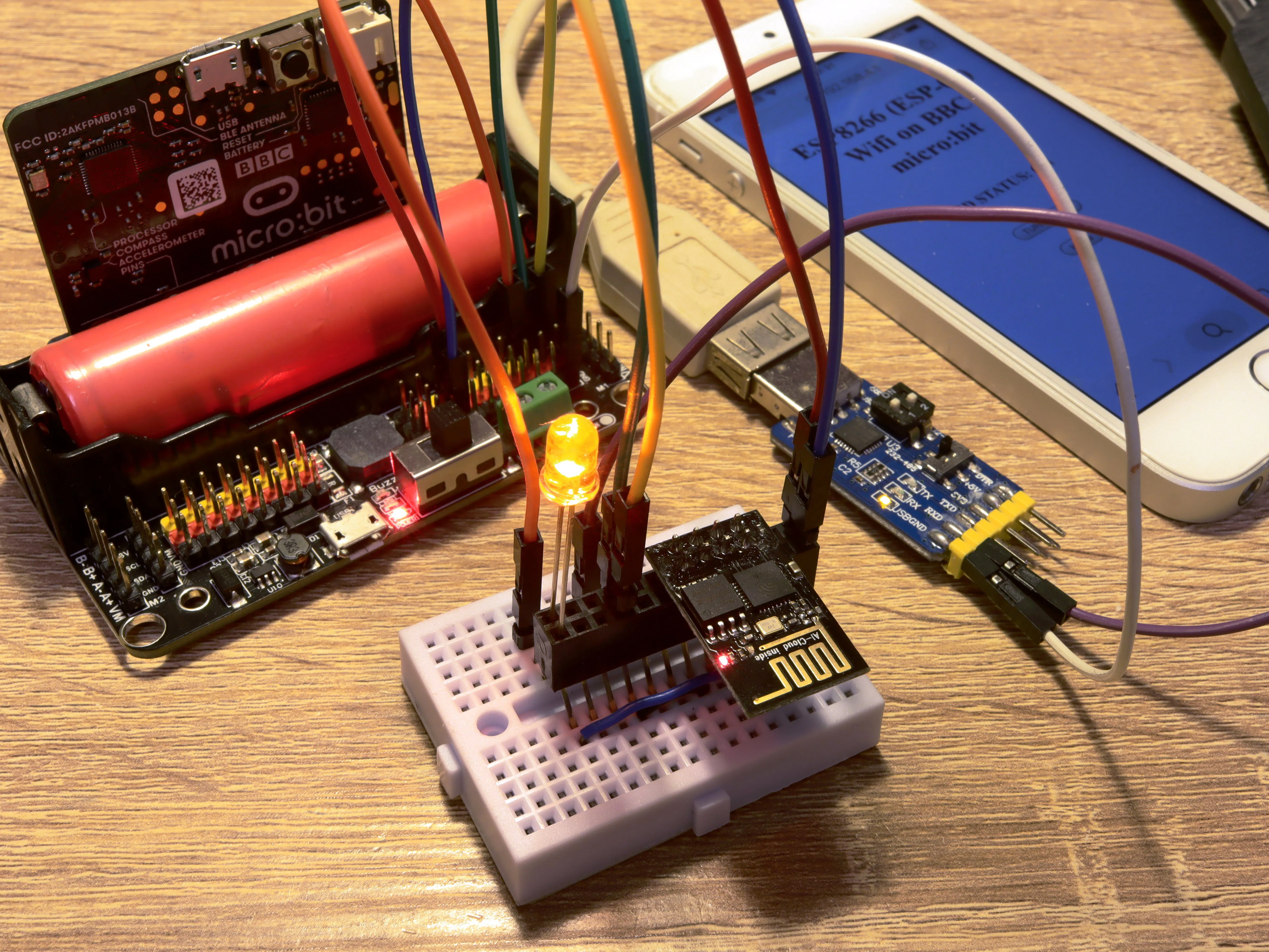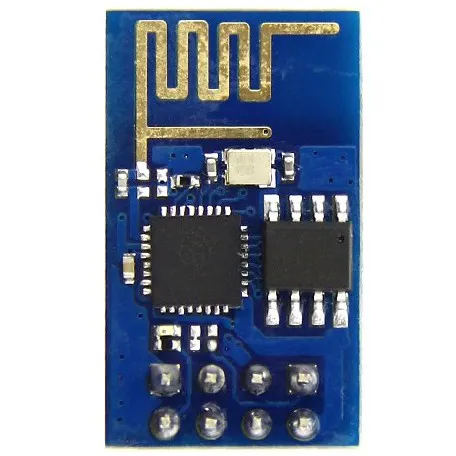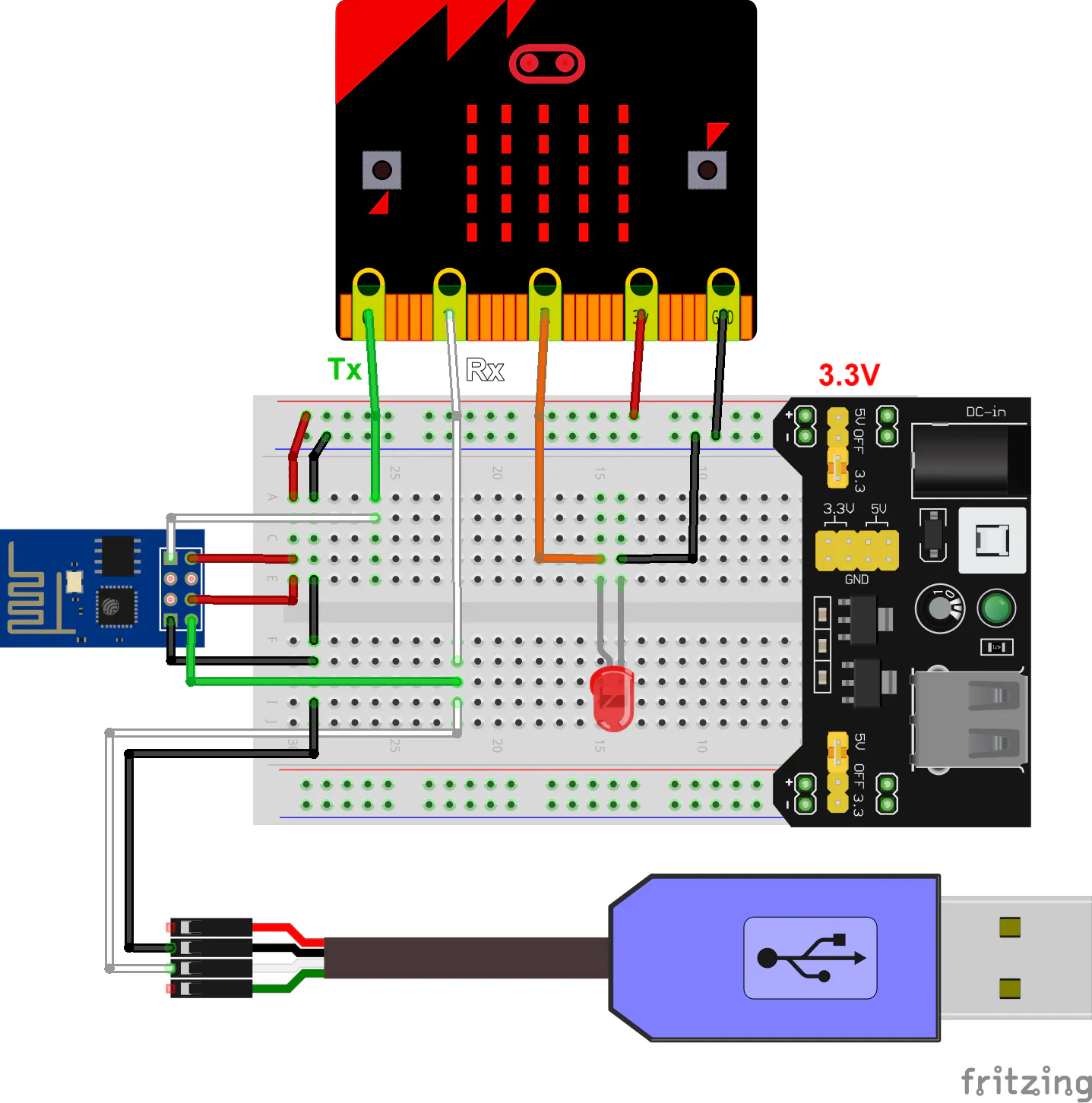Published © CC BY-NC

WiFi Web Server on BBC micro:bit and ESP-01 (ESP8266)

Create a micro:bit web server via AT commands which can respond to web browser requests over WiFi.

IntermediateFull instructions provided5,977Things used in this project

Hardware components

 BBC micro:bit
×1
 Edge Connector Breakout Board for BBC micro:bit Optional - you can use any micro:bit edge connectors/motor boards/expansion boards. Or you can use crocodile clip cables.
×1Espressif ESP8266 ESP-01 Both ESP-01 and ESP-01S works. Make sure that it has factory firmware.
×1
 USB-to-TTL cable/module Optional. You need this to read serial responses of the ESP-01 on your computer.
×1LED (generic) Also optional depends on what you want to control on micro:bit
×1×1
 3.3V external power source Capable for at least 200-400mA. You can also use special micro:bit edge connectors/motor boards/expansion boards which has strong 3.3v output.
×1Jumper wires (generic)
×1

Software apps and online servicesMicrosoft MakeCode

Schematics

Connect ESP-01 to micro:bit and 3.3v power

The micro:bit uses P0 and P1 as serial port, and P2 to a LED. The ESP-01 requires 3.3v power with about 200-400mA.Code

micro:bit + ESP-01 (ESP8266) Web Server Demo

Typescript
Open MakeCode editor for micro:bit (https://makecode.microbit.org), create a new project, click "JavaScript" on the top and paste the code into it.
// ESP8266 ESP-01 Wifi control via AT commands on BBC micro:bit
// by Alan Wang
// v0.02

// user settings
const WIFI_MODE: number = 2 // 1 = STA (station, connect to wifi router); 2 = AP (make itself an access point)
const Tx_pin: SerialPin = SerialPin.P0 // To Rx pin of ESP-01
const Rx_pin: SerialPin = SerialPin.P1 // To Tx pin of ESP-01
const LED_pin: DigitalPin = DigitalPin.P2 // pin for LED control
const SSID_1: string = "your_wifi_ssid" // wifi router ssid for station mode
const PASSWORD_1: string = "your_wifi_password" //wifi router password for station mode
const SSID_2: string = "ESP8266" // AP server ssid for AP mode
const PASSWORD_2: string = "microbit" // AP password for AP mode (at least 8 characters)

let LED_status: number = 0
let serial_str: string = ""

// initialize LED
pins.digitalWritePin(LED_pin, 0)
// redirect serial port
serial.redirect(Tx_pin, Rx_pin, 115200) // older ESP8266s may use 51200 or 9600...
// restore settings
sendAT("AT+RESTORE", 1000)
// reset
sendAT("AT+RST", 1000)
// set wifi mode
sendAT("AT+CWMODE=" + WIFI_MODE)
if (WIFI_MODE == 1) {
// join wifi router
sendAT("AT+CWJAP=\"" + SSID_1 + "\",\"" + PASSWORD_1 + "\"")
let result: boolean = wait_for_response("OK")
if (!result) control.reset()
} else if (WIFI_MODE == 2) {
// setup AP with 1 channels and authenticate mode = 4 (WPA_WPA2_PSK)
sendAT("AT+CWSAP=\"" + SSID_2 + "\",\"" + PASSWORD_2 + "\",1,4", 1000)
}
// allow multiple connections
sendAT("AT+CIPMUX=1")
//start web server
sendAT("AT+CIPSERVER=1,80")
// display IP (you will need this in STA mode; in AP mode it would be default 192.168.4.1)
sendAT("AT+CIFSR")
// startup completed
basic.showIcon(IconNames.Yes)

// process HTTP request
while (true) {
// read and store 200 characters from serial port
if (serial_str.length > 200) {
serial_str = serial_str.substr(serial_str.length - 200)
}
if (serial_str.includes("+IPD") && serial_str.includes("HTTP")) {
// got a HTTP request
let client_ID: string = serial_str.substr(serial_str.indexOf("IPD") + 4, 1)
let GET_pos: number = serial_str.indexOf("GET")
let HTTP_pos: number = serial_str.indexOf("HTTP")
let GET_command: string = serial_str.substr(GET_pos + 5, (HTTP_pos - 1) - (GET_pos + 5))
let GET_success: boolean = false
// handle GET command
switch (GET_command) {
case "": // request 192.168.x.x/
GET_success = true
break
case "LED": // request 192.168.x.x/LED
GET_success = true
LED_status = 1 - LED_status
pins.digitalWritePin(LED_pin, LED_status)
break
}
// output HTML
let HTML_str: string = getHTML(GET_success)
// send HTML to user
sendAT("AT+CIPSEND=" + client_ID + "," + (HTML_str.length + 2))
sendAT(HTML_str, 1000)
// close connection
sendAT("AT+CIPCLOSE=" + client_ID)
serial_str = ""
}
}

// write AT command with CR+LF ending
function sendAT(command: string, waitTime: number = 100) {
serial.writeString(command + "\u000D\u000A")
basic.pause(waitTime)
}

// generate HTML
function getHTML(normal: boolean): string {
let LED_statusString: string = ""
let LED_buttonString: string = ""
let web_title: string = "ESP8266 (ESP-01) Wifi on BBC micro:bit"
let html: string = ""
html += "HTTP/1.1 200 OK\r\n" // HTTP response
html += "Content-Type: text/html\r\n"
html += "Connection: close\r\n\r\n"
html += "<!DOCTYPE html>"
html += "<html>"
html += "<link rel=\"icon\" href=\"data:,\">"
html += "<title>" + web_title + "</title>"
html += "<meta name=\"viewport\" content=\"width=device-width, initial-scale=1\">" // mobile view
html += "<body>"
html += "<div style=\"text-align:center\">"
html += "<h1>" + web_title + "</h1>"
html += "<br>"
// generate status text
if (normal) {
if (LED_status) {
LED_statusString = "ON"
LED_buttonString = "TURN IT OFF"
} else {
LED_statusString = "OFF"
LED_buttonString = "TURN IT ON"
}
html += "<h3>LED STATUS: " + LED_statusString + "</h3>"
html += "<br>"
// generate buttons
html += "<input type=\"button\" onClick=\"window.location.href=\'LED\'\" value=\"" + LED_buttonString + "\">"
html += "<br>"
} else {
}
html += "<br>"
html += "<input type=\"button\" onClick=\"window.location.href=\'/'\" value=\"Home\">"
html += "</div>"
html += "</body>"
html += "</html>"
return html
}

// for wifi connection
function wait_for_response(str: string): boolean {
let result: boolean = false
let time: number = input.runningTime()
while (true) {
if (serial_str.length > 200) {
serial_str = serial_str.substr(serial_str.length - 200)
}
if (serial_str.includes(str)) {
result = true
break
}
if (input.runningTime() - time > 300000) break
}
return result
}

Credits

Alan Wang

14 projects • 21 followers
I make microbit, Arduino, ESP8266 and maybe Raspberry Pi stuff.